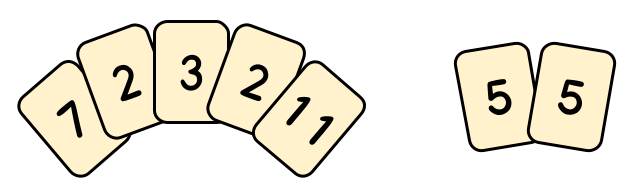시간 제한메모리 제한제출정답맞힌 사람정답 비율
45 초 (추가 시간 없음) 1024 MB (추가 메모리 없음)36151542.857%

## 문제

You are playing a new solitaire game called Prime Time. You are given a deck of cards, and each card has a prime number written on it. Multiple cards may have the same number.

Your goal is to divide the cards into two groups in such a way that the sum of the numbers in the first group is equal to the product of the numbers in the second group. Each card must belong to exactly one of the two groups, and each group must contain at least one card. The sum or product of a group that consists of a single card is simply the number on that card.For example, in the image above, the left group has cards whose sum is 25 and the right group has cards whose product is 25. Therefore, this is a valid split into groups.

Your score is the sum of the numbers in the first group (which is equal to the product of the numbers in the second group), or 0 if you cannot split the cards this way at all. What is the maximum score you can achieve?

## 입력

The first line of the input gives the number of test cases, T. T test cases follow. The first line of each test case contains a single integer M, representing the number of distinct prime numbers in your deck. Each of the next M lines contains two values: Pi and Ni, representing that you have exactly Ni cards with the prime Pi written on them.

Note that the total number of cards in your deck is the sum of all Nis.

## 출력

For each test case, output one line containing Case #x: y, where x is the test case number (starting from 1) and y is the maximum score you can achieve.

## 제한

• 1 ≤ T ≤ 100.
• 1 ≤ M ≤ 95. (Note that there are exactly 95 distinct primes between 2 and 499)
• 2 ≤ Pi ≤ 499, for all i.
• Each Pi is prime.
• Pi < Pi+1, for all i. (The primes are given in strictly increasing order)
• 1 ≤ Ni, for all i.

## Test Set 1 (7점)

• 2 ≤ N1 + N2 + ⋯ + NM ≤ 10.

## Test Set 2 (13점)

• 2 ≤ N1 + N2 + ⋯ + NM ≤ 100.

## Test Set 3 (15점)

• 2 ≤ N1 + N2 + ⋯ + NM ≤ 1015.

## 예제 입력 1

4
5
2 2
3 1
5 2
7 1
11 1
1
17 2
2
2 2
3 1
1
2 7


## 예제 출력 1

Case #1: 25
Case #2: 17
Case #3: 0
Case #4: 8


## 힌트

In Sample Case #1, the optimal split is: 11+2+7+3+2=5⋅5. Another split is also possible: 5+7+3+2+5=11⋅2, but it gives a lower score.

In Sample Case #2, note that cards with the same number can be placed in different groups.

## 채점 및 기타 정보

• 예제는 채점하지 않는다.